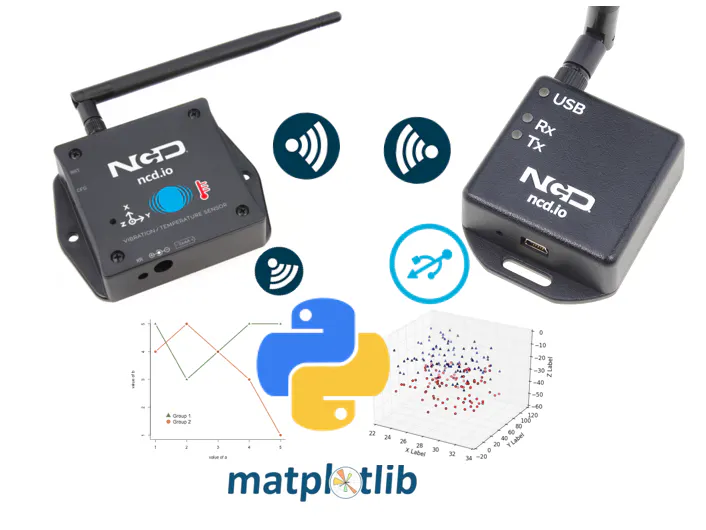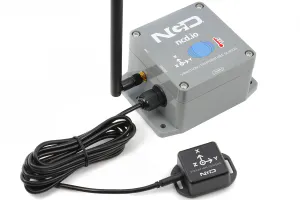Published

# 2D & 3D Live Graph Monitoring Wireless Vibration Sensor

Stepping forward core development which monitors the Wireless Vibration sensor data and plot on the graph in 2D & 3D axis using Python.

IntermediateFull instructions provided4 hours250## Things used in this project

### Hardware componentsNational Control Devices Industrial Wireless Vibration And Temperature Sensor
×1
 National Control Devices Xbee Coordinator
×1

## Code

### 3D axis Plotting Basic Live Graph

Python
```import numpy as np
import matplotlib.pyplot as plt
from mpl_toolkits.mplot3d import Axes3D
import matplotlib.animation as animation
import serial

seru = serial.Serial('COM6', 115200)
x = []
y = []
z = []
fig = plt.figure()
def update_lines(num):
#calling sensor function
rmsX,rmsY,rmsZ = vib_sense()
#Creating Numpy array and appending the values
vib_x= np.array(rmsX)
x.append(vib_x)
vib_y= np.array(rmsY)
y.append(vib_y)
vib_z= np.array(rmsZ)
z.append(vib_z)
print(x)
print(y)
print(z)
ax.clear()
#Limit the Graph
ax.set_xlim3d(0, 100)
ax.set_ylim3d(0, 100)
ax.set_zlim3d(0, 100)
#for line graph
graph = ax.plot3D(x,y,z,color='orange',marker='o')
#For Scatter
# graph = ax.scatter3D(x,y,z,color='orange',marker='o')
return graph

def vib_sense():
while True:
if(s == 126):
if(s == 127):
if(s== 8):
rms_x = ((s*65536)+(s*256)+s)/1000
rms_y = ((s*65536)+(s*256)+s)/1000
rms_z = ((s*65536)+(s*256)+s)/1000
'''
max_x = ((s*65536)+(s*256)+s)/1000
max_y = ((s*65536)+(s*256)+s)/1000
max_z = ((s*65536)+(s*256)+s)/1000
min_x = ((s*65536)+(s*256)+s)/1000
min_y = ((s*65536)+(s*256)+s)/1000
min_z = ((s*65536)+(s*256)+s)/1000
ctemp = ((s*256)+s)
battery = ((s*256)+s)
voltage = 0.00322*battery
'''
return rms_x,rms_y,rms_z

# Creating the Animation object
ani = animation.FuncAnimation(fig, update_lines, frames=200, interval=5, blit=False)
plt.show()
```

### 2D Live graph Wireless Vibration Sensor

Python
```import datetime as dt
import matplotlib.pyplot as plt
import matplotlib.animation as animation
import serial

seru = serial.Serial('COM6', 115200)

# Create figure for plotting
fig = plt.figure()

# Initialize globally
xs = []
ys = []
y2 = []
y3 = []

# Animate Function
def animate(i, xs, ys,y2,y3):
rmsX,rmsY,rmsZ = vib_sense();
print(rmsX);
print(rmsY);

#rounding off the figure upto 2 decimal format
rms = round(rmsX, 2)
rms1 = round(rmsY, 2)
rms2 = round(rmsZ, 2)

# Add x and y to lists
xs.append(dt.datetime.now().strftime('%H:%M:%S.%f'))
ys.append(rms)
y2.append(rms1)
y3.append(rms2)

# Limit x and y lists to 50 items
xs = xs[-50:]
ys = ys[-50:]
y2 = y2[-50:]
y3 = y3[-50:]

# Draw x and y lists
ax1.clear()
ax1.plot(xs, ys, label='RMS X', marker='o')
ax1.plot(xs, y2, label='RMS Y',marker='o')
ax1.plot(xs, y3, label='RMS Z',marker='o')

# Format plot
plt.xticks(rotation=60, ha='right')

plt.title('RMS Values for Vibration')
plt.ylabel('axis in mg')
plt.xlabel('Time')
plt.legend(loc='upper right')

# vibration Sensor Data
def vib_sense():
while True:
if(s == 126):
if(s == 127):
if(s== 8):
rms_x = (((s*65536)+(s*256)+s)& 0xffff)/100
rms_y = (((s*65536)+(s*256)+s)& 0xffff)/100
rms_z = (((s*65536)+(s*256)+s)& 0xffff)/100
max_x = (((s* 65536)+(s*256)+s)& 0xffff)/100
max_y = (((s*65536)+(s*256)+s)& 0xffff)/100
max_z = (((s*65536)+(s*256)+s)& 0xffff)/100
min_x = (((s*65536)+(s*256)+s)& 0xffff)/100
min_y = (((s*65536)+(s*256)+s)& 0xffff)/100
min_z = (((s*65536)+(s*256)+s)& 0xffff)/100
ctemp = (((s*256)+s)& 0xffff)
battery = ((s*256)+s)
voltage = 0.00322*battery
rmsValueX = 0;
rmsValueY = 0;

return rms_x,rms_y,rms_z

ani = animation.FuncAnimation(fig, animate, fargs=(xs, ys, y2, y3), interval=500)
plt.show()
```

## Credits

### Varul Jain

12 projects • 11 followers
Beginner, Creator, IOT Developer, working with IOT Prototypes